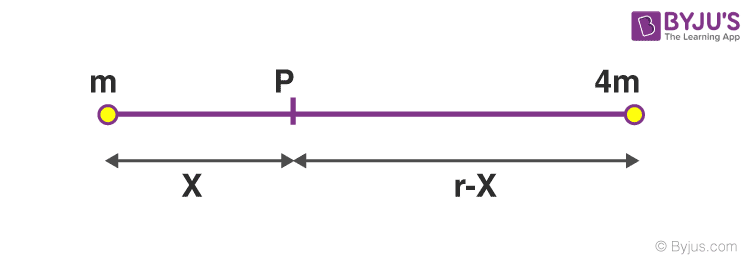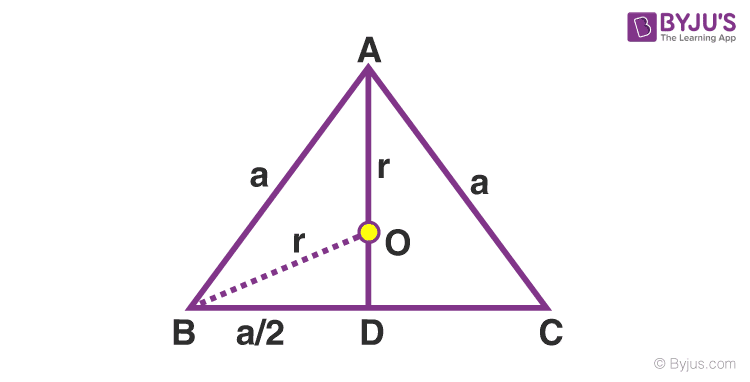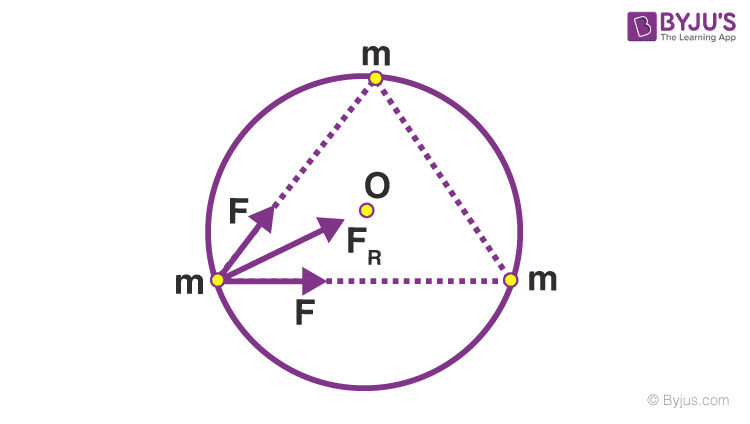# JEE Main Gravitation Previous Year Questions with Solutions

Gravitation is a natural phenomenon. It is the force of attraction between any two objects with mass or energy. Gravitational force that brings all the objects in the universe like planets, stars and galaxies together. The tides in the ocean are caused due to the gravitational force of attraction of the moon. But in most of the cases, the force is too weak to be observed due to the very large distance of separation. The effect of gravity reduces as the objects move away from each other. The weight of physical objects on earth is given as the product of its mass and the acceleration due to the gravity of earth. Read More.

This force of attraction was first observed by Sir Isaac Newton and was presented as Newton’s law of gravitation in the year 1680. However, gravitation can generally exist in two main instances.

## JEE Main Previous Year Solved Questions on Gravitation

Q1: If the distance between the earth and the sun were half its present value, the number of days in a year would have been

(a) 64.5

(b) 129

(c) 182.5

(d) 730

Solution: From Kepler’s law, T2∝ R3

Therefore, $\left ( \frac{T_{2}}{T_{1}} \right )^{2} = \left ( \frac{R_{2}}{R_{1}} \right )^{3}$

T1 = 365 days , R1 =R , R2 = R/2

$\left ( \frac{T_{2}}{365} \right )^{2} = \left ( \frac{R/2}{R} \right )^{3}$

T22 = (365)2/8

T22 = 16,653

T2 = 129 days

Q2: The mass of a spaceship is 1000 kg. It is to be launched from the earth’s surface out into free space. The value of ‘g’ and ‘R’ (radius of the earth) is 10 m/s2 and 6400 km respectively. The required energy for this work will be

(a) 6.4 x 1010 Joules

(b) 6.4 x 1011 Joules

(c) 6.4 x 108 Joules

(d) 6.4 x 109 Joules

Solution

The energy required is given by = GMm/R

= gR2 x m/R (∵ g = GM/R2)

= mgR

= 1000 x 10 x 6400 x 103

= 64 x 109 J

= 6.4 x 1010 J

Answer: (a) 6.4 x 1010 Joules

Q3: An artificial satellite moving in a circular orbit around the earth has a total (K.E. + P.E.) energy E0. Its potential energy is

(a) – E0

(b) 1.5 E0

(c) 2 E0

(d) E0

Solution

Total Energy, E0 = – GMm/2r

Potential Energy, U = -GMm/r = 2E0

Q4: The height at which the acceleration due to gravity becomes g/9 (where g = the acceleration due to gravity on the surface of the earth) in terms of R, the radius of the earth, is

(a) R/2

(b) R/3

(c) 2R

(d) 3R

Solution

Acceleration due to gravity at a height “h” is given by

g’ = g (R/R+h)2

Here,

g is the acceleration due to gravity on the surface

R is the radius of the earth

As g’ is given as g/9, we get

g/9 = g(R/R+h)2

⅓ = R/R+h

h=2R

Q5: A simple pendulum has a time period T1 when on the earth’s surface, and T2 when taken to a height R above the earth’s surface, where R is the radius of the earth. The value of T2/T1 is

(a) 1

(b) 3

(c) 4

(d) 2

Solution

The time period of a simple pendulum = $2\pi \sqrt{\frac{l}{g}}$

On the surface of earth g = GM/R2

At a height R above the earth g = GM/(2R)2

The time period on the surface of the earth T1 = $2\pi \sqrt{\frac{l(R)^{2}}{GM}}$

The time period on the surface of the earth T2 = $2\pi \sqrt{\frac{l(2R)^{2}}{GM}}$

T2/T1 = 2

Q6: A geostationary satellite orbits around the earth in a circular orbit of radius 36,000km. Then, the time period of a spy satellite orbiting a few hundred km above the earth’s surface (R =6,400km) will approximately be

(a) (l/2)hr

(b) 1 hr

(c) 2 hr

(d) 4 hr

Solution

According to Kepler’s law, T2∝ R3

Therefore, $\left ( \frac{T_{2}}{T_{1}} \right )^{2} = \left ( \frac{R_{2}}{R_{1}} \right )^{3}$

For a spy satellite time period is given by T1

R1 = 6400 km

For a geostationary satellite, T2 = 24hour

R2 = 36,000 km

$\left ( \frac{24}{T_{1}} \right )^{2} = \left ( \frac{36000}{6400} \right )^{3}$

(24/T1)2= 178

(24/T1) = 13.34

T1 = 24/13.34 ≈ 2hr

Q7: Two bodies of masses m and 4m are placed at a distance r. The gravitational potential at a point on the line joining them where the gravitational field is zero is

(a) -6Gm/r

(b) -9Gm/r

(c) Zero

(d) -4Gm/r

SolutionP is the point where the field is zero, and a unit mass is placed at P.

Applying Newton’s law of gravitation,

(Gm x 1)/x2 = (G4m x 1)/(r – x)2

x2/(r – x)2= ¼

x/(r – x)= ½

2x = r – x

x = r/3

Potential at the point P, V = – Gm/x – G(4m)/(r-x)

$V = -\frac{Gm}{r/3} – \frac{G(4m)}{(r-r/3)}$

V = -9Gm/r

Q8: A Binary star system consists of two stars A and B which have time periods TA and TB, radii RA and RB and masses MA and MB. Then

(a) TA ＞TB then RA ＞RB

(b) TA ＞TB then MA ＞MB

(c) (TA/TB)2 = (RA/RB)2

(d) TA = TB

Solution

Angular velocity of binary stars are the equal ωA = ωB

2π/TA = 2π /TB

⇒ TA = TB

Q9: Two particles of equal mass “m” go around a circle of radius R under the action of their mutual gravitational attraction. The speed of each particle with respect to their centre of mass is

(a) $\sqrt{Gm/R}$

(b) $\sqrt{Gm/4R}$

(c) $\sqrt{Gm/3R}$

(d) $\sqrt{Gm/2R}$

Solution

Gm2/4R2 = mV2/R

$V= \sqrt{Gm/4R}$

Answer: (b) $\sqrt{Gm/4R}$

Q10: A satellite is moving with a constant speed ‘V’ in a circular orbit about the earth. An object of mass “m” is ejected from the satellite such that it just escapes from the gravitational pull of the earth. At the time of its ejection, the kinetic energy of the object is

(a)1/2 mV2

(b) mV2

(c) 3/2 mV2

(d)2 mV2

Solution

Kinetic energy at the time of ejection = ½ mve2

Ve is the escape velocity = $\sqrt{2}$ x orbital velocity

Ve is the escape velocity = $\sqrt{2}v$

Kinetic energy at the time of ejection = ½ m( $\sqrt{2}v$)2

= mv2

Q11: Three particles, each of mass m, are situated at the vertices of an equilateral triangle of side length a. The only forces acting on the particles are their mutual gravitational forces. lt is desired that each particle moves in a circle while maintaining the original mutual separation a. Find the initial velocity that should be given to each particle and also the time period of the circular motion. (F = Gm1m2/r2)

Solution:

ABC is a equilateral triangle of side a

Each particle moves in a circle of radius r$r=\frac{2}{3}\times \sqrt{a^{2}-a^{2}/4}$ $r= a\sqrt{3}$ ——-(1)

1. To find vLet v= Initial velocity given to each particle.

For circular motion, centripetal force should be provided. It is provided by the gravitational force between two masses. Let F denote this force.

F = Gm2/a2——-(2)

Resultant force = $\sqrt{F^{2} + F^{2} +2F^{2}cos60^{0}}$

Resultant force = $\sqrt{3}F$

Resultant force = Centripetal force

$\sqrt{3}F$=mV2/r

$V^{2}=\frac{\sqrt{3}Fr}{m}=\frac{\sqrt{3}}{m}\times \left ( \frac{Gm^{2}}{a^{2}} \right )\left ( \frac{a}{\sqrt{3}} \right ) = Gm/a$ $V=\sqrt{\frac{Gm}{a}}$

2. To find time period of circular motion(T)

T = 2πr/V = $2\pi (a/\sqrt{3})\times \sqrt{a/Gm}=2\pi \sqrt{a^{3}/3Gm}$

therefore ,$T=2\pi \sqrt{a^{3}/3Gm}$

Answer: $V=\sqrt{\frac{Gm}{a}}$ and $T=2\pi \sqrt{a^{3}/3Gm}$

Q12: What is the minimum energy required to launch a satellite of mass m from the surface of a planet of mass M and radius R in a circular orbit at an altitude of 2R?

(a) 5GmM/6R

(b) 2GmM/3R

(c) GmM/2R

(d) GmM/3R

Solution

Energy of the satellite on the surface of the Earth E1 = -GMm/R

Energy at a distance 2R is given by E2 = -GMm/3R + ½mvo2

E2 = -GMm/3R + ½m[GM/3R]

E2 = -GmM/6R

E2 -E1 = (-GmM/6R) – ( -GMm/R ) = 5GmM/6R

Q13: Two satellites S1 and S2 revolve around a planet in coplanar circular orbits in the same sense. Their periods of revolution are 1 hour and 8 hours, respectively. The radius of the orbit of S1 is 104 km. When S2 is closest to S1, find the angular speed of S2 as actually observed by an astronaut in S1

Solution:

Angular velocity ω = 2π/T

Here T = 1 hour for S1,ω1=2π rad/hr

Similarly, for S2,ω2 = 2π

Given that they are rotating in the same sense. So, relative angular velocity = 2π – 2π/8= 7π/8

Q14: Suppose that the angular velocity of rotation of earth is increased. Then,as a consequence

(a) There will be no change in weight anywhere on the earth

(b) Weight of the object, everywhere on the earth, will increase

(c) Except at poles, weight of the object on the earth will decrease

(d) Weight of the object, everywhere on the earth, will decrease.

Solution

The effect of rotation of earth on acceleration due to gravity is given by g’ = g – ω2Rcos2Φ

Where Φ is latitude. There will be no change in gravity at poles as Φ = 900, at all points as ω increases g’ will decrease.

Answer: (c) Except at poles, weight of the object on the earth will decrease

Q15: A particle is moving with a uniform speed in a circular orbit of radius R in a central force inversely proportional to the nth power of R. If the period of rotation of the particle is T, then

(a) T ∝ R3/2

(b) T ∝ R(n/2)+1

(c) T ∝ R(n+1)/2

(d) T ∝ Rn/2

Solution

According to the question, central force is given by

Fc ∝ 1/Rn

Fc =k (1/Rn)

2R = k(1/Rn)

$m\frac{(2\pi )^{2}}{T^{2}}=k\frac{1}{R^{n+1}}$

Or T2 ∝ Rn+1

T ∝ R(n+1)/2